# GateLevel Minimization Chapter 3 The Map Method Twovariable

• Slides: 53Gate-Level Minimization Chapter 3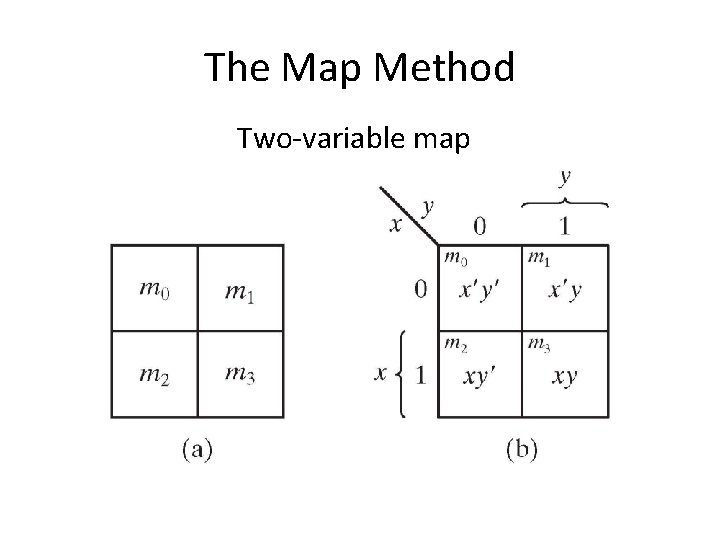The Map Method Two-variable map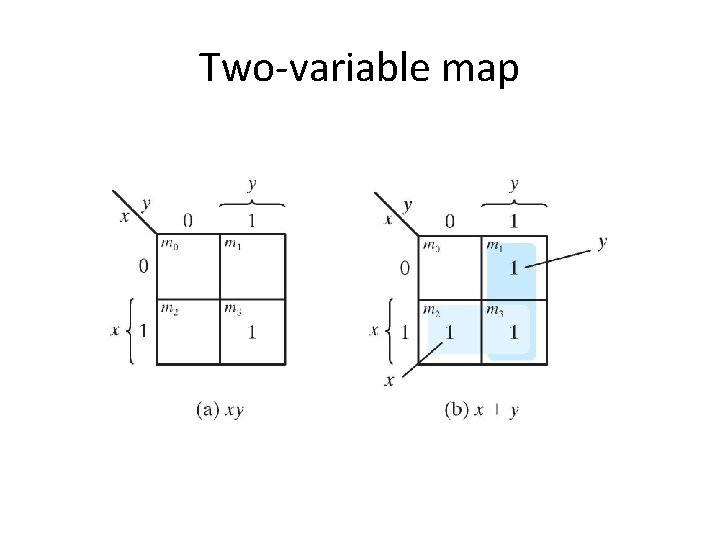Two-variable map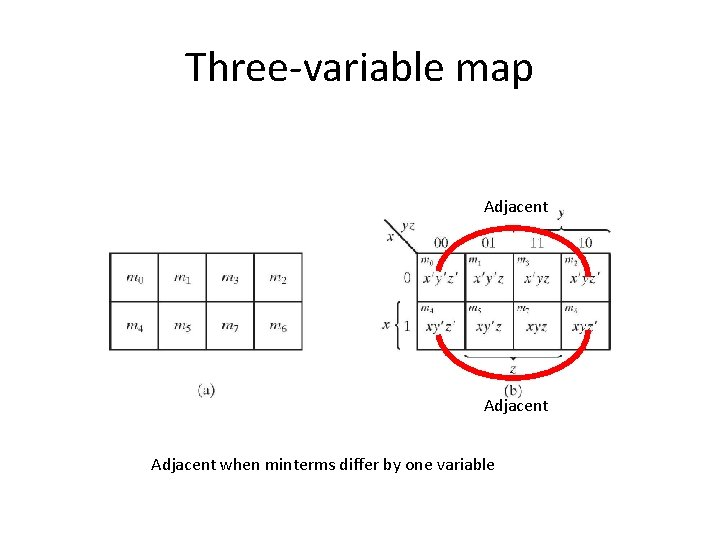Three-variable map Adjacent when minterms differ by one variable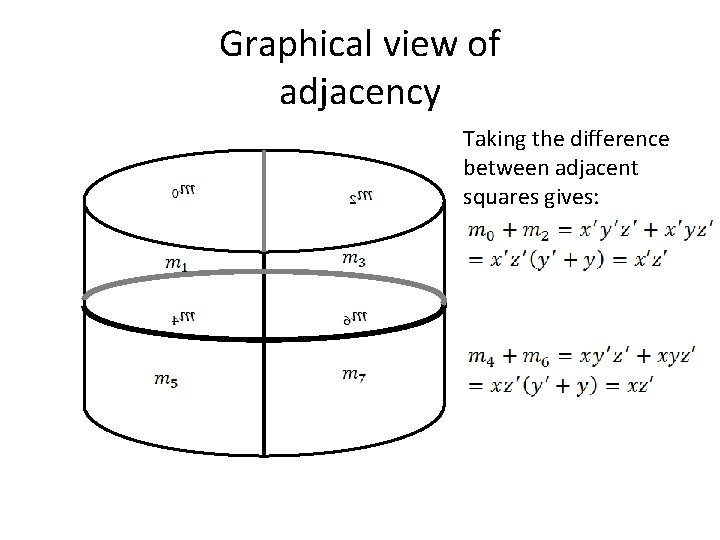Example 3. 1 Simplify the Boolean functionExample 3. 2 Simplify the Boolean FunctionThree variable map 00 0 1 01 11 10Three-variable map • The number of adjacent squares that may be combined must always represent a number that is a power of two: – One square represents one minterm which in this case gives a term with three literals – Two adjacent squares represent a term with two literals – Four adjacent squares represent a term with one literal – Eight adjacent squares encompass the entire map and produce a function equal to 1Three-variable map 00 0 1 01 11 10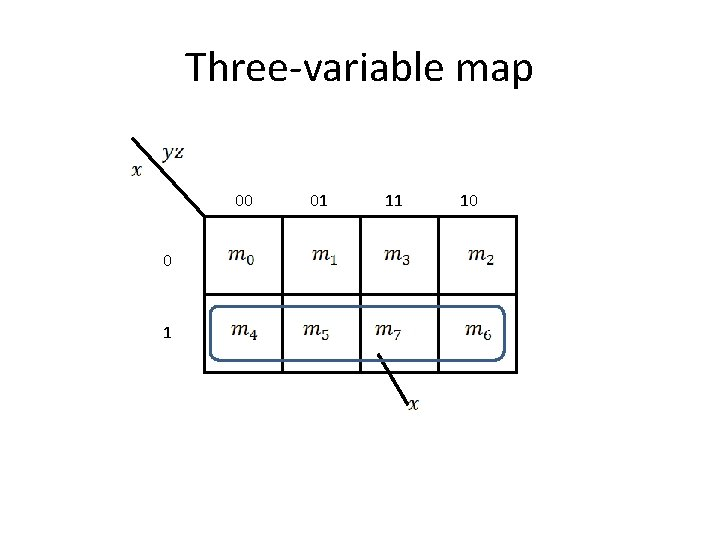Three-variable map 00 0 1 01 11 10Three-variable map 00 0 1 01 11 10Example 3. 3Example 3. 4 Let the Boolean Function (a) Express this function as a sum of minterms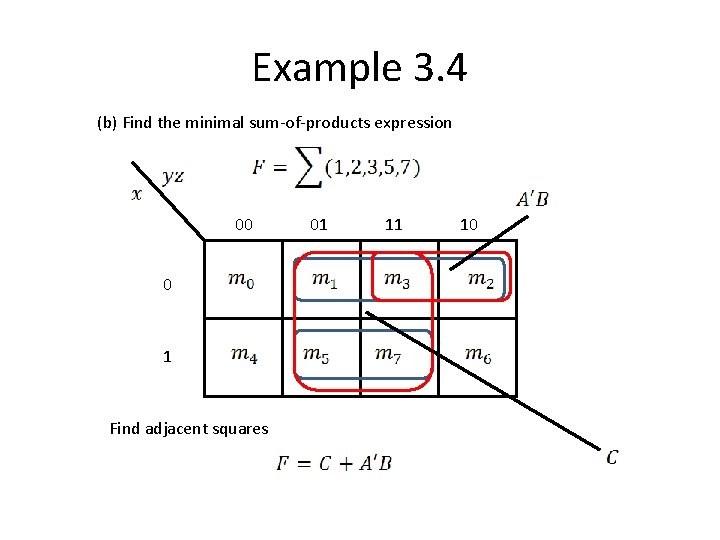Example 3. 4 (b) Find the minimal sum-of-products expression 00 0 1 Find adjacent squares 01 11 10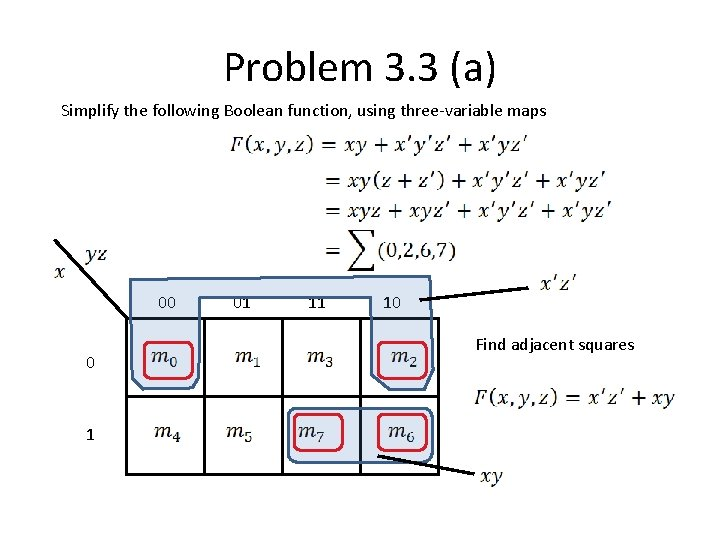Problem 3. 3 (a) Simplify the following Boolean function, using three-variable maps 00 0 1 01 11 10 Find adjacent squaresProblem 3. 3 (a) Another solution (without a three-variable map) Factorize the expression Voila!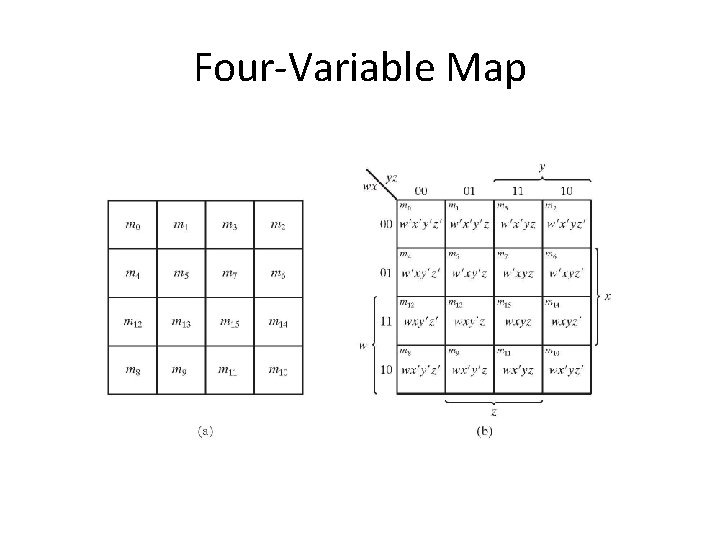Four-Variable Map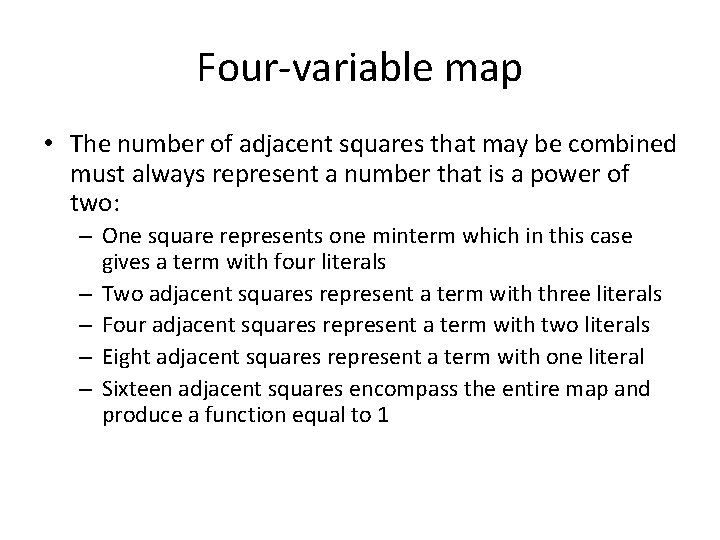Four-variable map • The number of adjacent squares that may be combined must always represent a number that is a power of two: – One square represents one minterm which in this case gives a term with four literals – Two adjacent squares represent a term with three literals – Four adjacent squares represent a term with two literals – Eight adjacent squares represent a term with one literal – Sixteen adjacent squares encompass the entire map and produce a function equal to 1Example 3. 5 Simplify the Boolean Function 00 01 11 10 00 1 1 1 01 1 1 1 10 1 1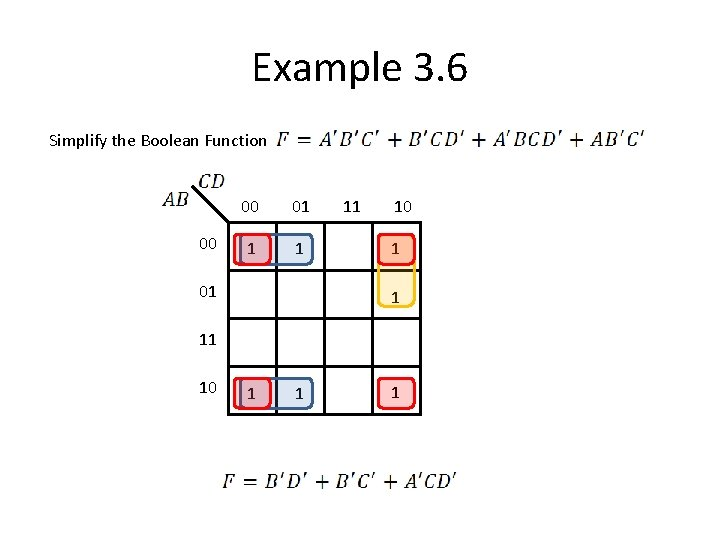Example 3. 6 Simplify the Boolean Function 00 00 01 1 1 01 11 10 1 1 1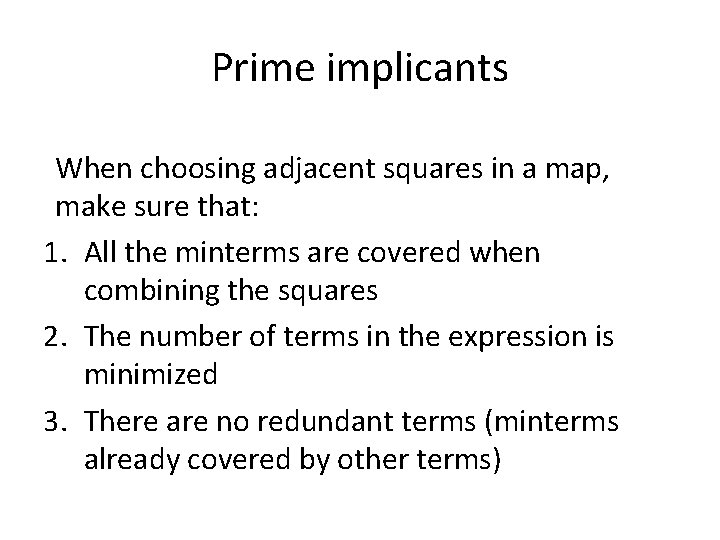Prime implicants When choosing adjacent squares in a map, make sure that: 1. All the minterms are covered when combining the squares 2. The number of terms in the expression is minimized 3. There are no redundant terms (minterms already covered by other terms)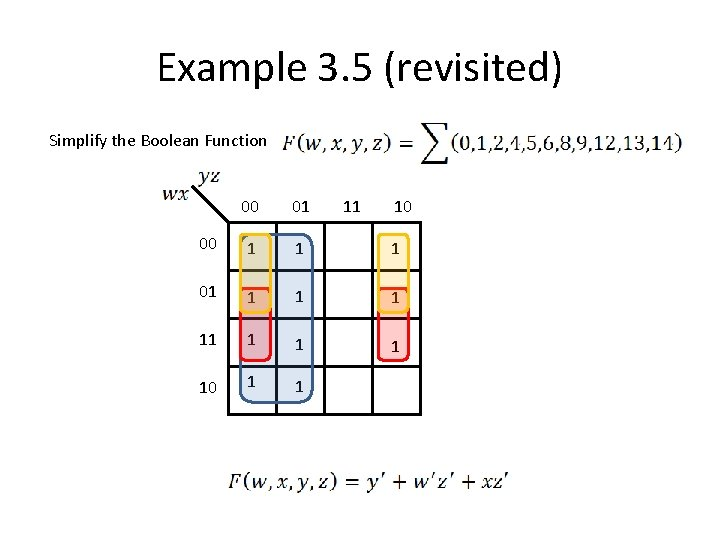Example 3. 5 (revisited) Simplify the Boolean Function 00 01 11 10 00 1 1 1 01 1 1 1 10 1 1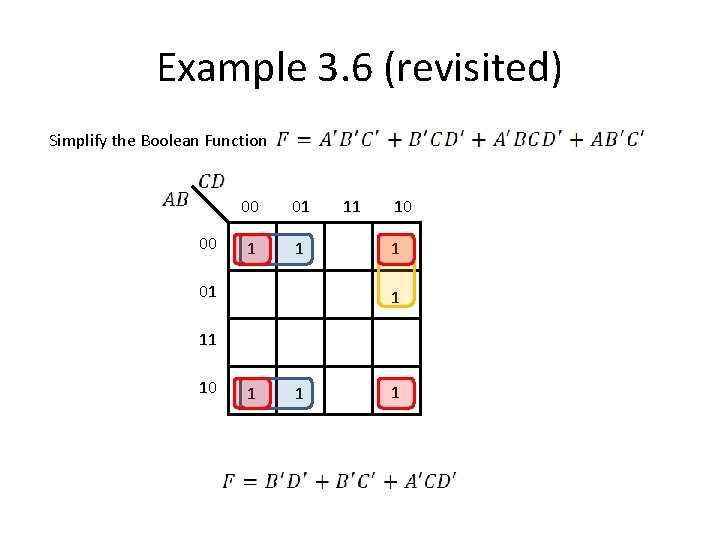Example 3. 6 (revisited) Simplify the Boolean Function 00 00 01 1 1 01 11 10 1 1 1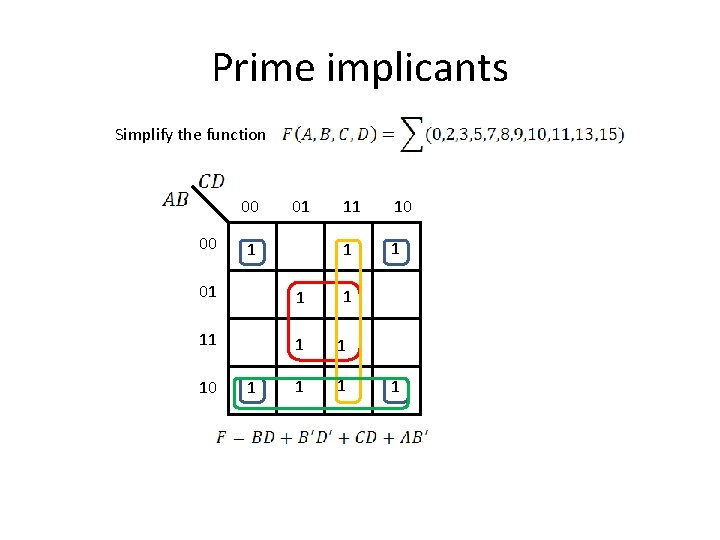Prime implicants Simplify the function 00 00 01 1 11 10 1 1 01 1 10 1 1 1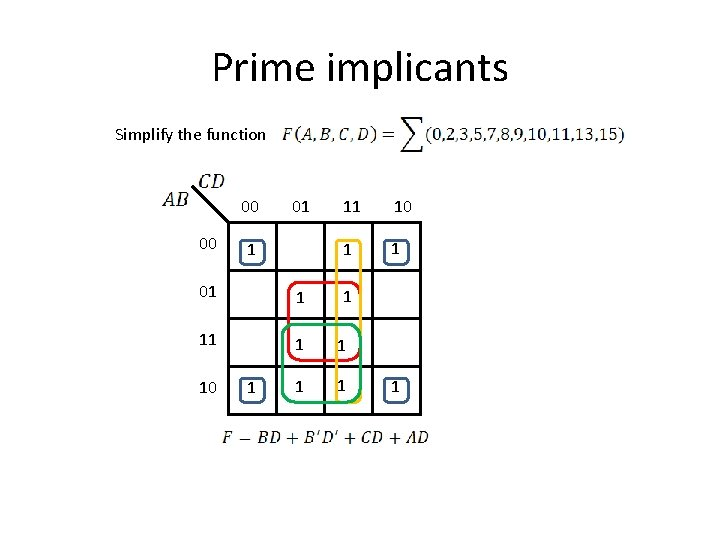Prime implicants Simplify the function 00 00 01 1 11 10 1 1 01 1 10 1 1 1Prime implicants Simplify the function 00 00 01 1 11 10 1 1 01 1 10 1 1 1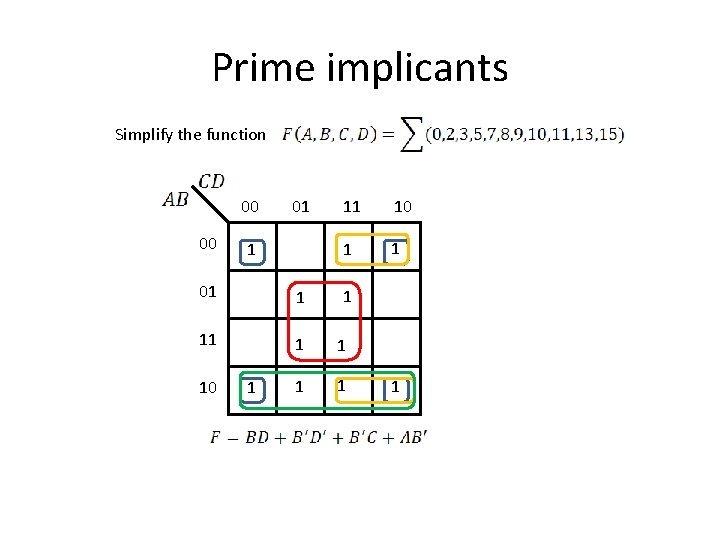Prime implicants Simplify the function 00 00 01 1 11 10 1 1 01 1 10 1 1 1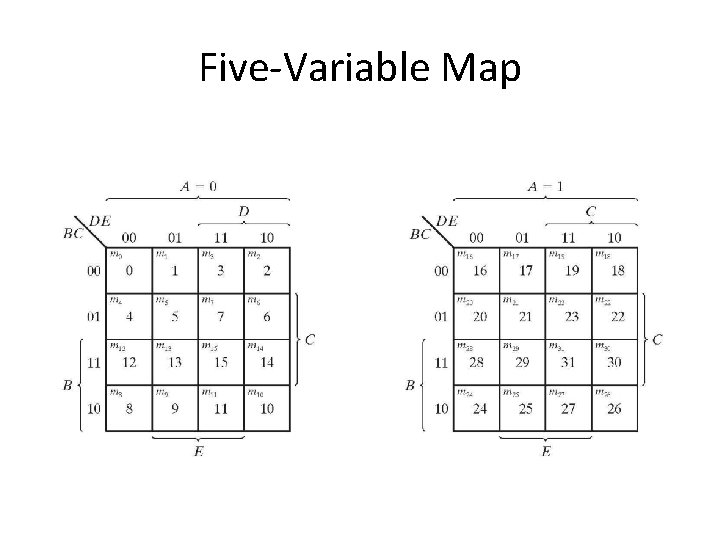Five-Variable Map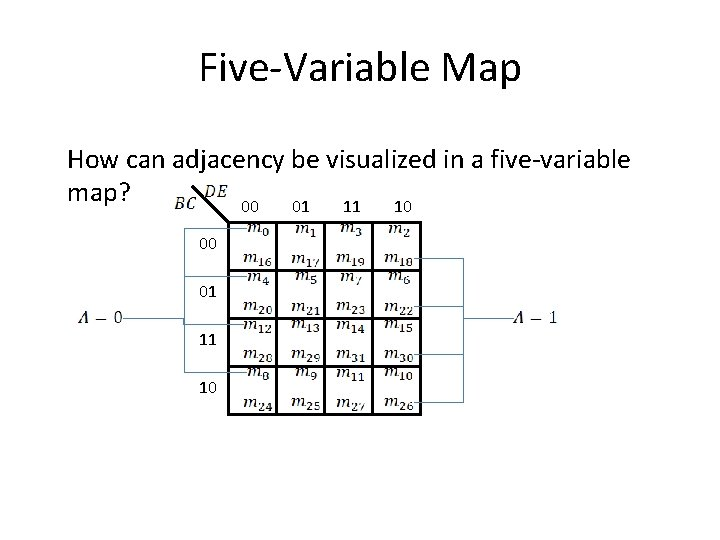Five-Variable Map How can adjacency be visualized in a five-variable map? 00 01 11 10Five-Variable MapFive-Variable Map Simplify the Boolean functionProduct-Of-Sums Simplification Take the squares with zeros and obtain the simplified complemented function Complement the above expression and use De. Morgan’s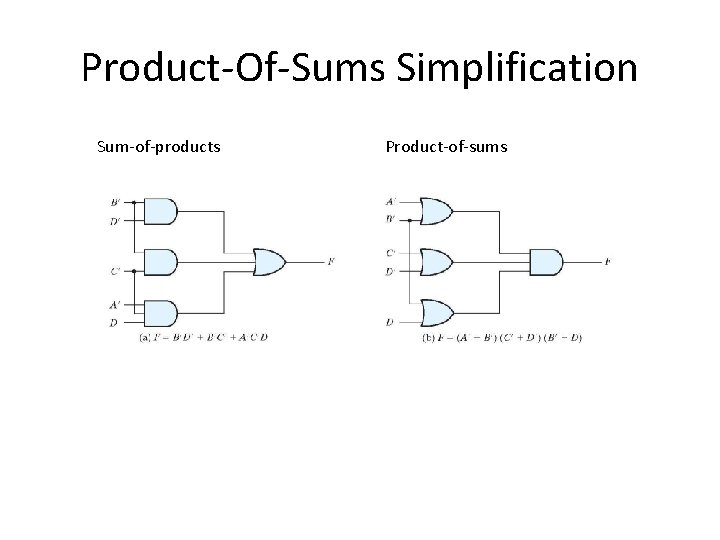Product-Of-Sums Simplification Sum-of-products Product-of-sumsDon’t-Care Conditions • Used for incompletely specified functions, e. g. BCD code in which six combinations are not used (1010, 1011, 1100, 1101, 1110, and 1111) • Those unspecified minterms are neither 1’s nor 0’s • Unspecified terms are referred to as “don’t care” and are marked as X • In choosing adjacent squares, don’t care squares can be chosen either as 1’s or 0’s to give the simplest expressionDon’t-Care Conditions Simplify the Boolean function which has the don’t care conditions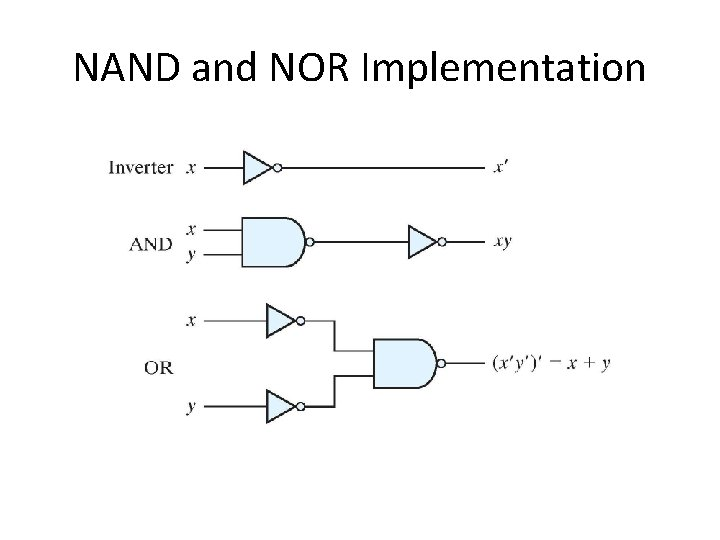NAND and NOR ImplementationNAND and NOR Implementation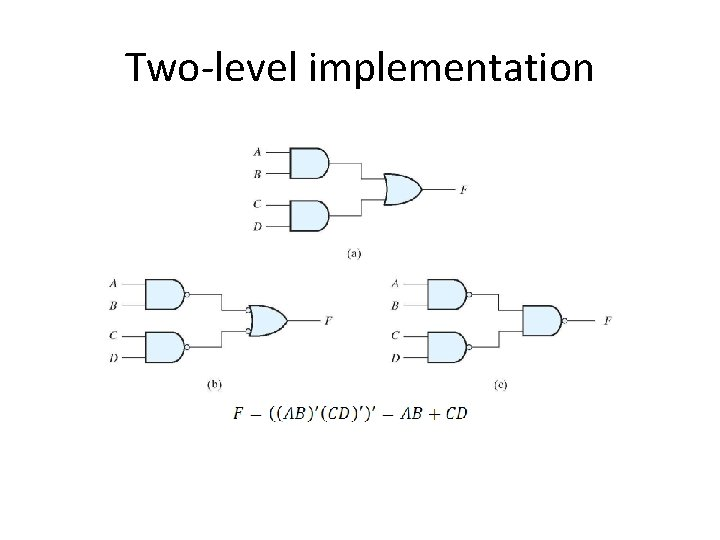Two-level implementation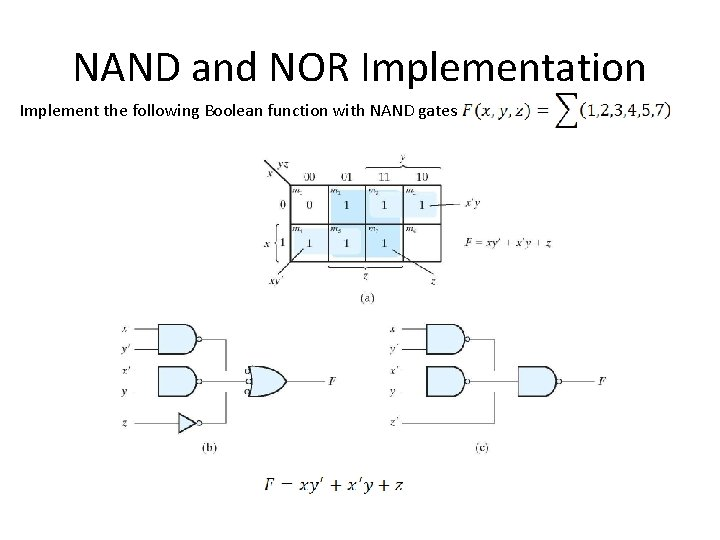NAND and NOR Implementation Implement the following Boolean function with NAND gatesMultilevel NAND circuits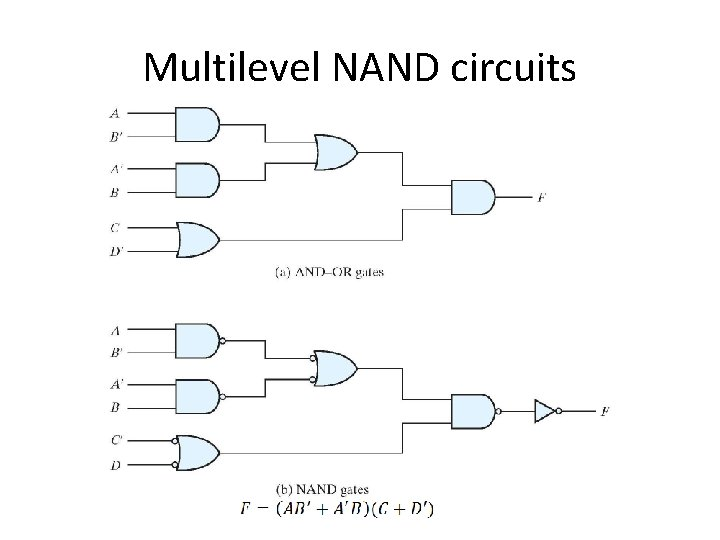Multilevel NAND circuits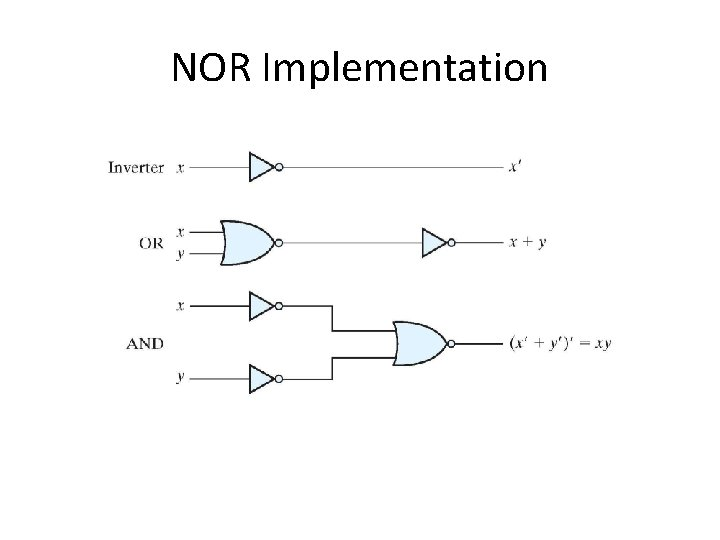NOR Implementation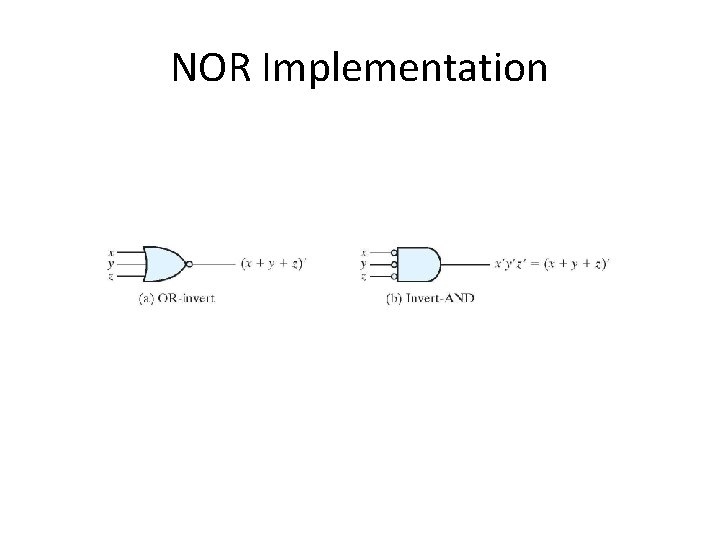NOR ImplementationNOR Implementation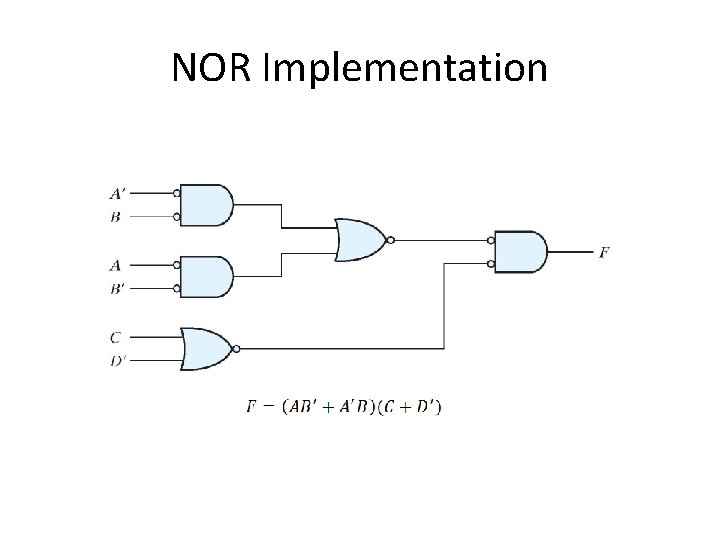NOR ImplementationExclusive OR Function Exclusive-OR or XOR performs the following logical operation Exclusive-NOR or equivalence performs the following logical operation Identities of the XOR operation XOR is commutative and associativeExclusive-OR implementations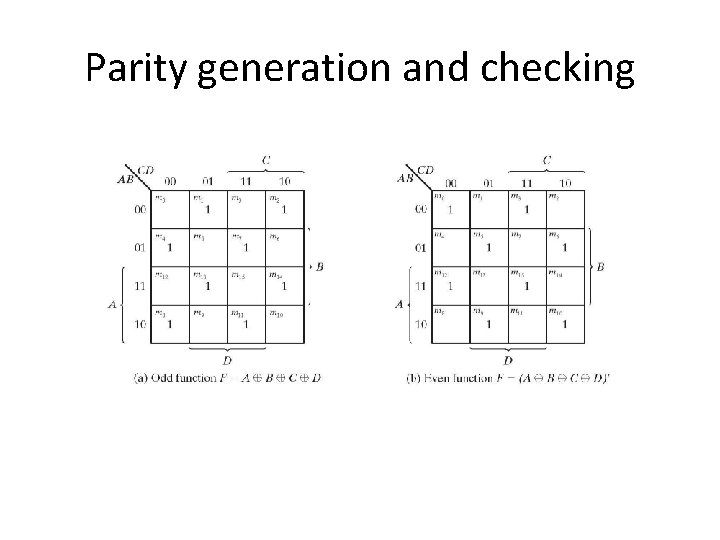Parity generation and checkingParity generation and checkingParity generation and checking